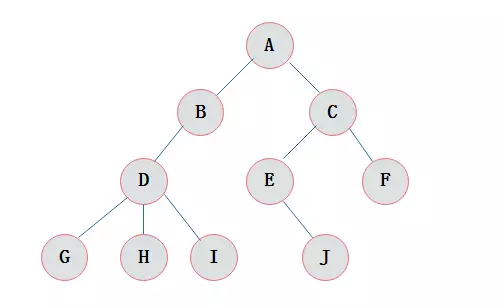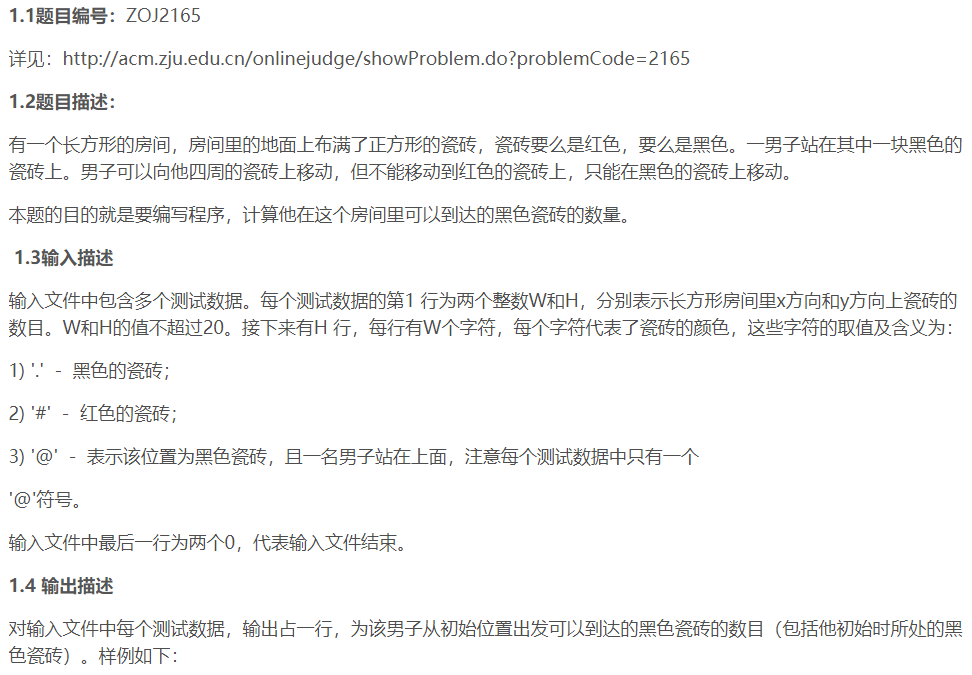# #2020寒假集训#搜索入门(BFS和DFS)代码笔记

wow好高级的名字！！！害，其实就是一种高级的遍历啦~

【DFS】全拼Depth First Search 有些题用DFS会TLE，但用BFS就能AC，时间复杂度较难分析【BFS】
（bool）ok函数：判断遍历到的点位是否符合题意，分别返回true和false
（int）sign变量：标记点位是否走/满足题意过
（int）ans变量：计算满足题意的点位数
（void）bfs函数：让main函数传入的值入队（这个值一般是全局变量定义，bfs无参数）

（int）main函数：传出初始位置+bfs()+打印输出结果

【DFS】
（bool）ok函数：判断遍历到的点位是否符合题意，分别返回true和false
（int）sign变量：标记点位是否走/满足题意过
（int）ans变量：计算满足题意的点位数
（void）dfs函数：让main函数传入的值入队（dfs有参数，因为要递归）

（int）main函数：初始化sign变量+循环遍历走过的被sign函数标记的点位+ans计数+打印输出结果

@其实通俗些来讲@
【广度优先搜索-横着看】顺序是A-B-C-D-E-F-G-H-I-J（先找兄弟结点）
【深度优先搜索-竖着看】顺序是A-B-D-G-D-H-I-D-B-A-C-E-J-E-C-F-C-A（先找子孙节点，勿忘倒回去）
@那么用代码实现的时候@
BFS广度】一般采用queue队列，高级些就是Priority_queue优先队列
DFS深度】一般采用递归或者

Red and Black
HZNU19training题源

Background
There is a rectangular room, covered with square tiles. Each tile is colored either red or black. A man is standing on a black tile. From a tile, he can move to one of four adjacent tiles. But he can’t move on red tiles, he can move only on black tiles.
Write a program to count the number of black tiles which he can reach by repeating the moves described above.

Input*
The input consists of multiple data sets. A data set starts with a line containing two positive integers W and H; W and H are the numbers of tiles in the x- and y- directions, respectively. W and H are not more than 20.
There are H more lines in the data set, each of which includes W characters. Each character represents the color of a tile as follows.
‘.’ - a black tile
‘#’ - a red tile
‘@’ - a man on a black tile(appears exactly once in a data set)

Output
For each data set, your program should output a line which contains the number of tiles he can reach from the initial tile (including itself).

Sample Input
6 9
…#.
…#

#@…#
.#…#.
11 9
.#…
.#.#######.
.#.#…#.
.#.#.###.#.
.#.#…@#.#.
.#.#####.#.
.#…#.
.#########.

11 6
…#…#…#…
…#…#…#…
…#…#…###
…#…#…#@.
…#…#…#…
…#…#…#…
7 7
…#.#…
…#.#…
###.###
…@…
###.###
…#.#…
…#.#…
0 0

Sample Output
45
59
6
13## - BFS广度优先搜索（又称宽度优先搜索or横向优先搜索）

#include<stdio.h>
#include<utility>
#include<string.h>
#include<queue>
using namespace std;
#define PII pair<int,int>//PII就是个宏定义代号啦
#define x first//define前面要带#，后面不能加；
#define y second//define格式：#define 标识符 字符串
const int N=1e2+10;
int n,m,sx,sy;
char s[N][N];
int use[N][N];//记录某个各自是否已经走过，1走过；0未走过
int Move=//实现四个方向的位移，注意不要用move全小写命名，可能报错
{
{-1,0},
{1,0},
{0,1},
{0,-1},
};
bool ok(int x,int y)//判断可走性
{
if(x<1||x>n||y<1||y>m) return false;//出界了不可走
if(s[x][y]=='#') return false;//红格子（#）不可走
if(use[x][y]) return false;//走过了不可走
return true;//前三条的return都没进行，那么就说明可走
}
void bfs()//广度优先搜索（也称作 宽度or横向 优先搜索）
{
memset(use,0,sizeof(use));
queue<PII> que;
que.push(make_pair(sx,sy));
use[sx][sy]=1;
int res=0;
while(!que.empty())//只要瘦新元素放进来，就能继续循环走位
{
PII u=que.front();//u是队列的首元素
que.pop();//移除队列首元素，但注意，已经记录在u里面了喔
res++;
for(int i=0;i<4;i++)
{
int nx=u.x+Move[i];
/*
Move的第二个下表中，0表示x坐标，1表示y坐标
i的1 2 3 4分别是往左 右 上 下
*/
int ny=u.y+Move[i];
if(ok(nx,ny))
{
use[nx][ny]=1;
que.push(PII(nx,ny));//把新元素放到队首
}
}
}
printf("%d\n",res);
}
int main()
{
while(~scanf("%d %d",&m,&n),m+n)
{
for(int i=1;i<=n;i++)
{
scanf("%s",s[i]+1);
/*
s[i]+1即本来是从s[i]开始存数据，现在从s[i]开始存数据
其实就是为了后续每个格子都能用直观的行列数做下标
第一行第一列的数就用1,1；而不是0,0
调用起来顺手些、舒服些
*/
}
for(int i=1;i<=n;i++)
{
for(int j=1;j<=m;j++)
{
if(s[i][j]=='@')//找到开始位置
{
sx=i;
sy=j;
}
}
}
bfs();//sx和xy是开始位置的横纵坐标
}
return 0;
}


## - DFS深度优先搜索（又称宽度优先搜索or横向优先搜索）

#include<stdio.h>
#include<utility>
#include<string.h>
using namespace std;
#define x first//define前面要带#，后面不能加；
#define y second//define格式：#define 标识符 字符串
const int N =1e2+10;
int m,n;//m是列数；n是行数
char s[N][N];
int use[N][N];//记录某个各自是否已经走过，1走过；0未走过
int Move=//定实现四个方向的位移
{
{-1,0},
{1,0},
{0,1},
{0,-1},
};
bool ok(int x,int y)//判断可走性
{
if(x<1||x>n||y<1||y>m) return false;//出界了不可走
if(s[x][y]=='#') return false;//红格子（#）不可走
if(use[x][y]) return false;//走过了不可走
return true;//前三条的return都没进行，那么就说明可走
}
void dfs(int x,int y)//深度优先搜索
{
if(s[x][y]=='#') return;
/*
void类型函数强制终止运行下面的语句时，可直接写return
void类型的函数无返回值
*/
if(use[x][y]) return;
use[x][y]=1;
for(int i=0;i<4;i++)
{
int nx=x+Move[i];
/*
Move的第二个下表中，0表示x坐标，1表示y坐标
i的1 2 3 4分别是往左 右 上 下
*/
int ny=y+Move[i];
if(ok(nx,ny)) dfs(nx,ny);
/*
递归走格子，这个格子能走就从这个格子开始再找能往哪走
递归回去判断新use记不记1
*/
}
}
int main()
{
while(~scanf("%d %d",&m,&n),m+n)//m+n确保它俩不同时为零，否则程序结束
{
memset(use,0,sizeof(use));
for(int i=1;i<=n;i++)
{
scanf("%s",s[i]+1);
/*
s[i]+1即本来是从s[i]开始存数据，现在从s[i]开始存数据
其实就是为了后续每个格子都能用直观的行列数做下标
第一行第一列的数就用1,1；而不是0,0
调用起来顺手些、舒服些
*/
}
for(int i=1;i<=n;i++)
{
for(int j=1;j<=m;j++)
{
if(s[i][j]=='@') dfs(i,j);
//遍历找到@就找到了起始位置，开始深度优先搜索
}
}
int res=0;//res记录走过的格子有几个
for(int i=1;i<=n;i++)
{
for(int j=1;j<=m;j++)
{
res+=use[i][j];//走过的是1，没走过的是0
}
}
printf("%d\n",res);
}
return 0;
}


N皇后问题
HZNU19training题源

Background

Input

Output

Sample Input
1
8
5
0

Sample Output
1
92
10

## - DFS深度优先搜索（又称宽度优先搜索or横向优先搜索）

#include<stdio.h>
#include<string.h>
using namespace std;
const int N=1e2+10;
int n,sign[N][N],res,ans[N];
/*
n为输入的棋盘尺寸、皇后数量
ans数组记录输入每个n的情况的答案
sign数组在标记棋盘位置上有没有皇后，有皇后了就要标记1
当新开一种情况遍历的时候，注意清空g数组中会影响之后判断的标记
*/
bool ok(int x,int y)
{
for(int i=1;i<=n;i++)
{
for(int j=1;j<=n;j++)
{
if(!(x==i&&y==j))
{//x,y是传入的位置，遍历其他位置，判断皇后能不能待在x,y这个位置
if(sign[i][j]==1)
{
if(i==x) return false;//同行
if(j==y) return false;//同列
if(i+j==x+y) return false;//同撇斜线
if(i-j==x-y) return false;//同捺斜线
}
}
}
}
return true;
}
void dfs(int cur)
{
if(cur==n+1)//如果cur已经超出了棋盘维度，说明每一行都有皇后了，完成任务
{
res++;//实现要求，算作一种摆放可能
/*
如果某一行每个位置都放不了皇后
那么i的遍历就会结束，函数结束
但注意只是那一行的函数结束
它只是说明第一行的某一个位置开始，完成不了皇后的放置
只有当第一行每个位置都确认完毕函数才真正结束
不然只是递归内部有出口了
*/
return;//强制退出函数
}
for(int i=1;i<=n;i++)
{
if(ok(cur,i))//判断地cur行第i列放皇后可不可行，找到可行的那一列
{
sign[cur][i]=1;
/*
从这个ok过了的位置开始放，那么得标记1说明有皇后了
在一个初始i产生的一系列递归里面，会一层层地多出标记1的位置
调用ok函数的时候就要判断是否冲突，判断可行性
*/
dfs(cur+1);
/*
一行找到皇后位置了就找下一行，看看下一行还有没有皇后能待的位置
在新的一行依旧要从左到右一整行都试一遍
*/
sign[cur][i]=0;
/*
一个初始i结束了，从下一个初始i开始
因为是新的分支情况，一切都得重来
但其实对于第n行，也可能有m种情况
这个时候就相当于，新开了一个m种之一的情况
那么上一个m之一的情况以后产生的标记都得清空
*/
}
}
}
int main()
{
memset(ans,-1,sizeof(ans));
while(scanf("%d",&n),n)
{//棋盘n*n，皇后有n个，n==0时终止
if(ans[n]!=-1)
{//重复输入同一个n的时候，节约时间直接调取答案，不要再遍历一遍啦！！！
printf("%d\n",ans[n]);
continue;
}
memset(sign,0,sizeof(sign));//给数组初始化的时候，sizeof括号里填写数组名
res=0;
dfs(1);
/*
从第一行开始遍历，一行一行找皇后，在函数内部递归(DFS)
用res在函数内部求和即可
*/
ans[n]=res;
printf("%d\n",ans[n]);
}
return 0;
}
`

©️2019 CSDN 皮肤主题: 1024 设计师: 上身试试